Next: 3.3.2 Gummel's Pentagon Up: 3.3 Examples Previous: 3.3 Examples

## 3.3.1 Homogeneous Semiconductor

As a first example homogeneously doped Si blocks with sidelength a = 10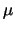m as shown in Fig. 3.8a were investigated. The doping levels were ND = 1017 cm-3 and NA = 1017 cm-3, respectively. Up to medium electric fields the DD and HD currents agree as one would expect. However, for extremely high-fields close to the breakdown voltage the difference was found to be 3%. This arises from the equilibrium contact condition for the carrier temperatures

 T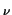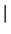contact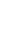= TL (3.67)

which dramatically violates the local energy balance equation by causing a strong gradient in the carrier temperature. As the electric field is constant and hence known at the contact one can derive a boundary condition from (3.36) which eliminates this discrepancy.

 Tcontact= TL +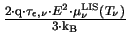(3.68)

The resulting electric fields and carrier temperatures for both contact models are shown in Fig. 3.9 and Fig. 3.10. Equation (3.68) results in a constant electric field and thus, via (3.36), in constant carrier temperatures. The conventional equilibrium contact model forces the hole temperature to be equal to the lattice temperature at the left contact. As the holes move from left to right, they pick up energy from the electric field and their temperature rises. As a consequence, the mobility reduces. To keep up the constant current density the electric field has to increase accordingly. However, for normal ohmic contacts the electric field at the contact is small, hence it is not necessary to use (3.68) except for this pathological situation.

The I-V curves for n-doped and p-doped semiconductors are shown in Fig. 3.11. In addition to the matching mobility models, the p-doped semiconductor was simulated using (3.41) with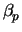= 1 in combination with (3.50) which is one of the most common errors. As can be seen, the error is intolerably large.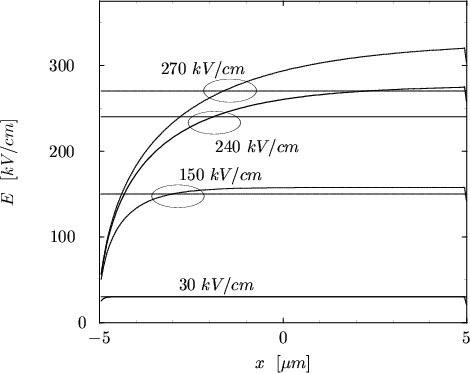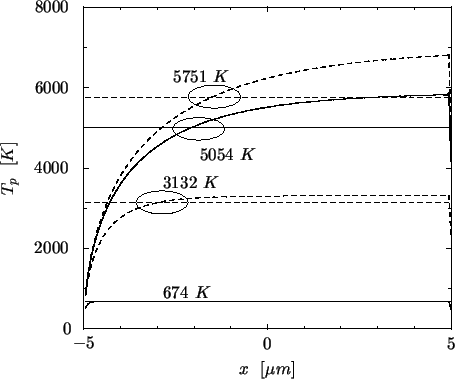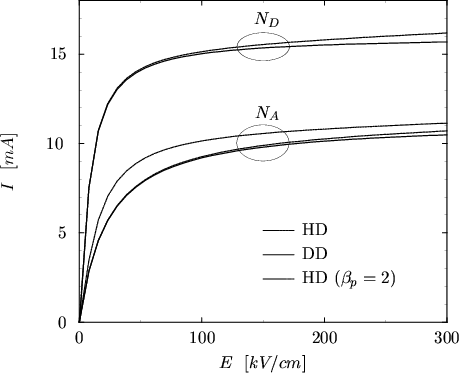Next: 3.3.2 Gummel's Pentagon Up: 3.3 Examples Previous: 3.3 Examples
Tibor Grasser
1999-05-31